xGudermannian functionEncyclopedia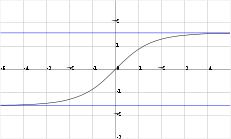The Gudermannian function, named after Christoph Gudermann
Christoph Gudermann
Christoph Gudermann was born in Vienenburg. He was the son of a school teacher and became a teacher himself after studying at the University of Göttingen, where his advisor was Karl Friedrich Gauss...

(1798–1852), relates the circular functions and hyperbolic function
Hyperbolic function
In mathematics, hyperbolic functions are analogs of the ordinary trigonometric, or circular, functions. The basic hyperbolic functions are the hyperbolic sine "sinh" , and the hyperbolic cosine "cosh" , from which are derived the hyperbolic tangent "tanh" and so on.Just as the points form a...

s without using complex numbers.

It is defined by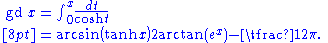Some related formulas don't quite work as definitions. For example, for real x,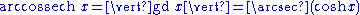. (See inverse trigonometric function
Inverse trigonometric function
In mathematics, the inverse trigonometric functions are the inverse functions of the trigonometric functions with suitably restricted domains .The notations sin−1, cos−1, etc...

s.)

The following identities hold: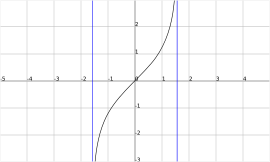The inverse
Inverse function
In mathematics, an inverse function is a function that undoes another function: If an input x into the function ƒ produces an output y, then putting y into the inverse function g produces the output x, and vice versa. i.e., ƒ=y, and g=x...

Gudermannian function, which is defined on the interval −π/2 < x < π/2, is given by(See inverse hyperbolic function
Inverse hyperbolic function
The inverses of the hyperbolic functions are the area hyperbolic functions. The names hint at the fact that they give the area of a sector of the unit hyperbola in the same way that the inverse trigonometric functions give the arc length of a sector on the unit circle...

s.)

The derivative
Derivative
In calculus, a branch of mathematics, the derivative is a measure of how a function changes as its input changes. Loosely speaking, a derivative can be thought of as how much one quantity is changing in response to changes in some other quantity; for example, the derivative of the position of a...

s of the Gudermannian and its inverse are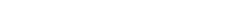The expression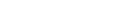defines the angle of parallelism
Angle of parallelism
In hyperbolic geometry, the angle of parallelism φ, also known as Π, is the angle at one vertex of a right hyperbolic triangle that has two asymptotic parallel sides. The angle depends on the segment length a between the right angle and the vertex of the angle of parallelism φ...

function in hyperbolic geometry
Hyperbolic geometry
In mathematics, hyperbolic geometry is a non-Euclidean geometry, meaning that the parallel postulate of Euclidean geometry is replaced...

.

## History

The function was introduced by Johann Heinrich Lambert
Johann Heinrich Lambert
Johann Heinrich Lambert was a Swiss mathematician, physicist, philosopher and astronomer.Asteroid 187 Lamberta was named in his honour.-Biography:...

in the 1760s at the same time as the hyperbolic functions. He called it the "transcendent angle," and it went by various names until 1862 when Arthur Cayley
Arthur Cayley
Arthur Cayley F.R.S. was a British mathematician. He helped found the modern British school of pure mathematics....

suggested it be given its current name as a tribute to Gudermann's work in the 1830s on the theory of special functions. Gudermann had published articles in Crelle's Journal
Crelle's Journal
Crelle's Journal, or just Crelle, is the common name for a mathematics journal, the Journal für die reine und angewandte Mathematik .- History :...

that were collected in Theorie der potenzial- oder cyklisch-hyperbolischen functionen (1833), a book which expounded sinh and cosh to a wide audience (under the guises of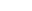and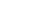).

The notation gd first appears on page 19 of the Philosophical Magazine
Philosophical Magazine
The Philosophical Magazine is one of the oldest scientific journals published in English. Initiated by Alexander Tilloch in 1798, in 1822 Richard Taylor became joint editor and it has been published continuously by Taylor & Francis ever since; it was the journal of choice for such luminaries as...

, vol. XXIV, where Cayley starts by calling gd. u the inverse of the integral of the secant function
Integral of the secant function
The integral of the secant function of trigonometry was the subject of one of the "outstanding open problems of the mid-seventeenth century", solved in 1668 by James Gregory. In 1599, Edward Wright evaluated the integral by numerical methods – what today we would call Riemann sums...

: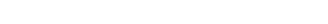and then derives "the definition" of the transcendent: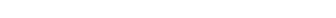observing immediately that it is a real function of u.

## Applications

The Gudermannian of the latitudinal
Latitude
In geography, the latitude of a location on the Earth is the angular distance of that location south or north of the Equator. The latitude is an angle, and is usually measured in degrees . The equator has a latitude of 0°, the North pole has a latitude of 90° north , and the South pole has a...

(due North/South) distance from the equator
Equator
An equator is the intersection of a sphere's surface with the plane perpendicular to the sphere's axis of rotation and containing the sphere's center of mass....

on a Mercator projection
Mercator projection
The Mercator projection is a cylindrical map projection presented by the Belgian geographer and cartographer Gerardus Mercator, in 1569. It became the standard map projection for nautical purposes because of its ability to represent lines of constant course, known as rhumb lines or loxodromes, as...

is the meridian arc
Meridian arc
In geodesy, a meridian arc measurement is a highly accurate determination of the distance between two points with the same longitude. Two or more such determinations at different locations then specify the shape of the reference ellipsoid which best approximates the shape of the geoid. This...

length, i.e. actual latitude on the globe.

The Gudermannian appears in a non-periodic solution of the inverted pendulum
Inverted pendulum
An inverted pendulum is a pendulum which has its mass above its pivot point. It is often implemented with the pivot point mounted on a cart that can move horizontally and may be called a cart and pole...

.

• Hyperbolic secant distribution
• Mercator projection
Mercator projection
The Mercator projection is a cylindrical map projection presented by the Belgian geographer and cartographer Gerardus Mercator, in 1569. It became the standard map projection for nautical purposes because of its ability to represent lines of constant course, known as rhumb lines or loxodromes, as...

• Tangent half-angle formula
• Tractrix
Tractrix
Tractrix is the curve along which a small object moves, under the influence of friction, when pulled on a horizontal plane by a piece of thread and a puller that moves at a right angle to the initial line between the object and the puller at an infinitesimal speed. It is therefore a curve of...

• Trigonometric identity